# AP Chemistry Practice Test 43

### Test Information12 questions18 minutes

1. Oxalic acid, H2C2O4, is a useful chemical for rust removal. A student prepared five oxalic acid samples by dissolving 0.9000 grams of oxalic acid in 100.00 mL of water and pipetting 10.00 mL samples of this solution into five separate beakers. Each of the samples was diluted with deionized water, and an appropriate indicator was added as an indicator. The samples were then titrated with standard 0.05000 M sodium hydroxide, NaOH, until the appearance of a permanent color change of the indicator indicated the endpoint of the titration. The following volumes were obtained. Molar mass of H2C2O4 = 90.04 g mol-1.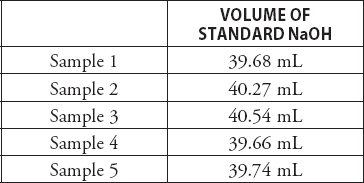The student calculated a concentration of approximately 0.20 M in each case. This is not the correct value. What is the most likely mistake that the student made?

2.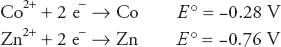Given the above standard reduction potentials, estimate the approximate value of the ΔG° for the following reaction:

Zn + Co2+ → Zn2+ + Co

`Questions 3-7 refer to the following information.pH versus volume of titrant added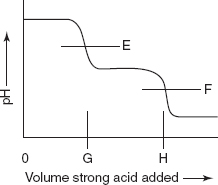The diagram above represents the idealized titration curve for the reaction of pure sodium carbonate, Na2CO3, with a strong acid such as hydrochloric acid, HCl. E and F represent the pH at the endpoints corresponding to the formation of HCO3- and H2CO3, respectively. G and H correspond to the quantity of acid required to reach the endpoints.`

3. A trial run used a sample of pure sodium carbonate. How does the volume of acid necessary to reach G from 0 compare to the volume of acid necessary to get from G to H?

4. The analysis of a sample contaminated with NaHCO3 gave slightly different results. How does the volume of acid necessary to reach G from 0 compare to the volume of acid necessary to get from G to H for the second sample?

5. How could a student determine if there was a strong acid or a strong base contaminant in the original sample?

6. In addition to water, what are the predominant species in solution at F?

7. At what point on the graph for the titration of pure sodium carbonate is the pH = pKa2 for carbonic acid?

8. Three steel containers hold gas samples. The containers are all the same size and at the same temperature. One container has 4.0 g of helium, another has 28.0 g of nitrogen, and the third has 44.0 g of carbon dioxide. Pick the FALSE statement from the following list:

9. When potassium perchlorate, KClO4, dissolves in water, the temperature of the resultant solution is lower than the initial temperature of the components. Which of the following conclusions may be related to this?

10. What is the reason that the lightest member of Group 15 does not follow the trend of the other members, which show that the boiling point decreases with decreasing atomic mass of the Group 15 element?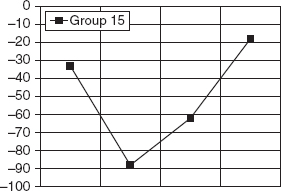The graph shows the variation of boiling point with Group number for the hydrogen compounds of the four lightest members of Group 15 on the periodic table (NH3, PH3, AsH3 and SbH3).

11.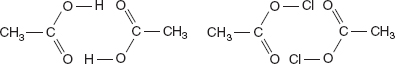A dimer consists of two closely associated molecules. In the gas phase, acetic acid tends to form dimers as illustrated on the left in the above diagram. Acetyl chloride, on the right in the above diagram, is not very efficient in forming dimers. Why is acetic acid better able to form dimers than acetyl chloride?

12.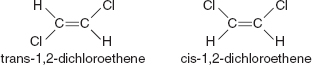Two compounds with the formula C2H2Cl2 appear in the above diagram. These two compounds are isomers. The molecules are planar and have the approximate structures shown in the diagram. The boiling point of trans-1, 2-dichloroethene is 47.5°C and the boiling point of cis-1,2-dichloroethene is 60.3°C. Which of the following best explains why cis-1,2-dichloroethene has a higher boiling point than its isomer, trans-1, 2-dichloroethene?